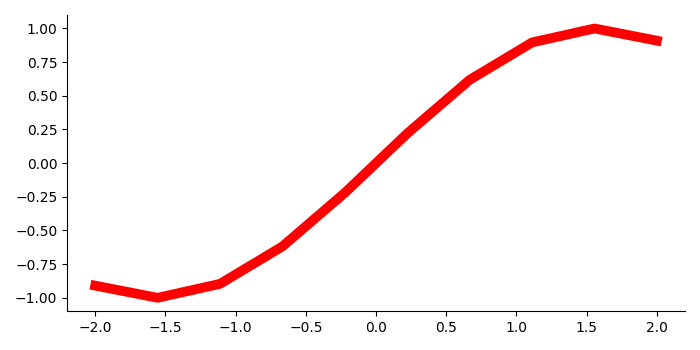# How to display only a left and bottom box border in Matplotlib?

To display or hide box border in matplotlib, we can use spines (value could be right, left, top or bottom) and set_visible() method to set the visibility to True or False.

## Steps

• Create x and y data points using numpy.

• Create a figure and add a set of subplots using subplots() method.

• Plot x and y data points using plot() method, where linewidth=7 and color=red.

• Set visibility as True for left and bottom, and False for top and right.

• To display the figure, use show() method.

## Example

import numpy as np
from matplotlib import pyplot as plt
plt.rcParams["figure.figsize"] = [7.00, 3.50]
plt.rcParams["figure.autolayout"] = True
x = np.linspace(-2, 2, 10)
y = np.sin(x)
fg, ax = plt.subplots()
ax.plot(x, y, lw=7, c='red')
ax.spines['right'].set_visible(False)
ax.spines['top'].set_visible(False)
ax.spines['left'].set_visible(True)
ax.spines['bottom'].set_visible(True)
plt.show()

## Output